Test: Graph Theory

# Test: Graph Theory

Test Description

## 10 Questions MCQ Test Network Theory (Electric Circuits) | Test: Graph Theory

Test: Graph Theory for Electrical Engineering (EE) 2022 is part of Network Theory (Electric Circuits) preparation. The Test: Graph Theory questions and answers have been prepared according to the Electrical Engineering (EE) exam syllabus.The Test: Graph Theory MCQs are made for Electrical Engineering (EE) 2022 Exam. Find important definitions, questions, notes, meanings, examples, exercises, MCQs and online tests for Test: Graph Theory below.
Solutions of Test: Graph Theory questions in English are available as part of our Network Theory (Electric Circuits) for Electrical Engineering (EE) & Test: Graph Theory solutions in Hindi for Network Theory (Electric Circuits) course. Download more important topics, notes, lectures and mock test series for Electrical Engineering (EE) Exam by signing up for free. Attempt Test: Graph Theory | 10 questions in 30 minutes | Mock test for Electrical Engineering (EE) preparation | Free important questions MCQ to study Network Theory (Electric Circuits) for Electrical Engineering (EE) Exam | Download free PDF with solutions
 1 Crore+ students have signed up on EduRev. Have you?
Test: Graph Theory - Question 1

### Consider the following circuits :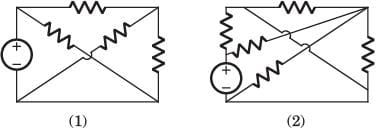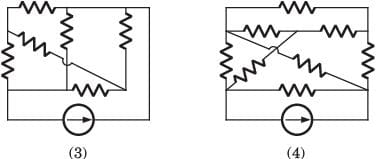The planner circuits are

Detailed Solution for Test: Graph Theory - Question 1

The circuit 1 and 2 are redrawn as below. 3 and 4 can not be redrawn on a plane without crossing other branch.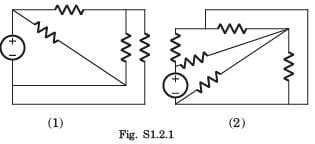Test: Graph Theory - Question 2

### Consider the following graphs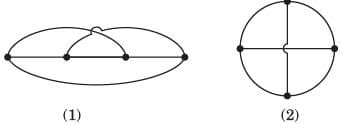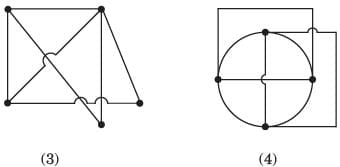Non-planner graphs are

Detailed Solution for Test: Graph Theory - Question 2

Other three circuits can be drawn on plane without crossing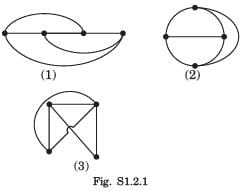Test: Graph Theory - Question 3

### A graph of an electrical network has 4 nodes and 7 branches. The number of links l, with respect to the chosen tree, would be

Detailed Solution for Test: Graph Theory - Question 3

l = b - ( n - 1) = 4.

Test: Graph Theory - Question 4

For the graph shown in fig. P.1.1.4 correct set is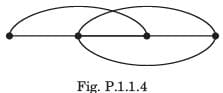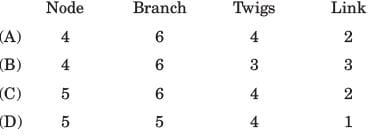Detailed Solution for Test: Graph Theory - Question 4

There are 4 node and 6 branches.
t = n - 1 = 3, l = b - n + 1 = 3

Test: Graph Theory - Question 5

A tree of the graph shown in fig. P.1.2.5 is​Detailed Solution for Test: Graph Theory - Question 5

From fig. it can be seen that afhg is a tree of given graph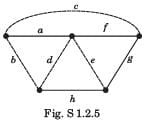Test: Graph Theory - Question 6

Branch current and loop current relation are expressed in matrix form as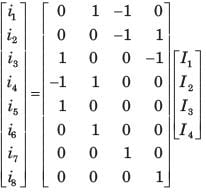where irepresent branch current and Ik loop current. The number of independent node equation are

Detailed Solution for Test: Graph Theory - Question 6

Number of branch = 8
Number of twigs = 8 - 4 = 4
Number of twigs =number of independent node equation.

Test: Graph Theory - Question 7

If the number of branch in a network is b, the number of nodes is n and the number of dependent loop is l, then the number of independent node equations will be

Detailed Solution for Test: Graph Theory - Question 7

The number of independent node equation are n - 1.

Test: Graph Theory - Question 8

Branch current and loop current relation are expressed in matrix form as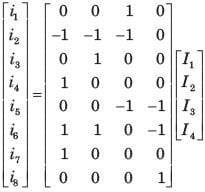where ij represent branch current and Ik loop current.
Q. The rank of incidence matrix is

Detailed Solution for Test: Graph Theory - Question 8

Number of branch b=8
Number of link l = 4
Number of twigs t = b - l = 4
rank of matrix = n - 1 = t = 4

Test: Graph Theory - Question 9

Branch current and loop current relation are expressed in matrix form aswhere ij represent branch current and Ik loop current.
Q. The directed graph will be

Detailed Solution for Test: Graph Theory - Question 9

We know the branch current and loop current are related as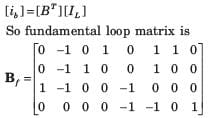f-loop 1 include branch (2, 4, 6, 7) and direction of branch–2 is opposite to other (B only).

Test: Graph Theory - Question 10

A network has 8 nodes and 5 independent loops.The number of branches in the network is

Detailed Solution for Test: Graph Theory - Question 10

l = b - ( n - 1)
⇒ 5= b - 7, b = 12

## Network Theory (Electric Circuits)

23 videos|21 docs|27 tests
 Use Code STAYHOME200 and get INR 200 additional OFF Use Coupon Code
Information about Test: Graph Theory Page
In this test you can find the Exam questions for Test: Graph Theory solved & explained in the simplest way possible. Besides giving Questions and answers for Test: Graph Theory , EduRev gives you an ample number of Online tests for practice

## Network Theory (Electric Circuits)

23 videos|21 docs|27 tests